Function Repository Resource:

# TraceTree

Generate a tree of all expressions in an evaluation chain

Contributed by: Ian Ford (Wolfram Research)
 ResourceFunction["TraceTree"][expr] generates a tree of all expressions used in the evaluation of expr. ResourceFunction["TraceTree"][expr,form] includes only those expressions that match form. ResourceFunction["TraceTree"][expr,s] includes all evaluations that use transformation rules associated with the symbol s.

## Details and Options

In general, form in ResourceFunction["TraceTree"][expr,form] is compared both with each complete expression that is evaluated and with the tag associated with an transformation rule used in the evaluation.
ResourceFunction["TraceTree"][expr,lhsrhs] picks out expressions that match lhs, then replaces them with rhs in the tree returned.
All expressions in the tree returned by ResourceFunction["TraceTree"] are wrapped in HoldForm.
ResourceFunction["TraceTree"] returns a Tree object. Each non-leaf subtree corresponds to a single evaluation chain, which contains the sequence of forms found for a particular expression. Subtrees have branches that give histories of subsidiary evaluations.
A rounded rectangular node is used for the first form in an evaluation chain. An unlabeled circular node is used as a placeholder when the first form is not included.
Rectangular nodes are used for all forms in an evaluation chain after the first form. An unlabeled square node is used as a placeholder when the last form is not included.
Options for ResourceFunction["TraceTree"] include:
 MatchLocalNames True whether to allow x to stand for x\$nnn TraceAbove False whether to show evaluation chains that contain the chain containing form TraceBackward False whether to show expressions preceding form in the evaluation chain TraceDepth Infinity how many levels of nested evaluations to include TraceForward False whether to show expressions following form in the evaluation chain TraceOff None forms within which to switch off tracing TraceOn _ forms within which to switch on tracing TraceOriginal False whether to look at expressions before their heads and arguments are evaluated
During the execution of ResourceFunction["TraceTree"], the settings for the form argument, and for the options TraceOn and TraceOff, can be modified by resetting the values of the global variables \$TracePattern, \$TraceOn, and \$TraceOff, respectively.

## Examples

### Basic Examples (2)

Trace each step in an evaluation:

 In:=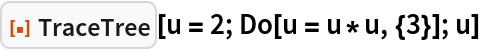Out=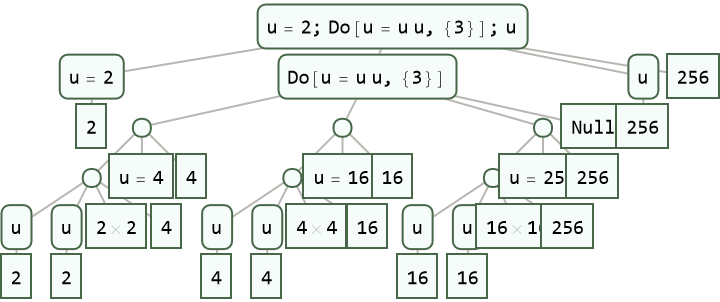Trace only the computations with head Times:

 In:=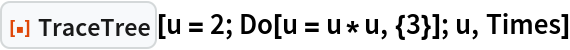Out=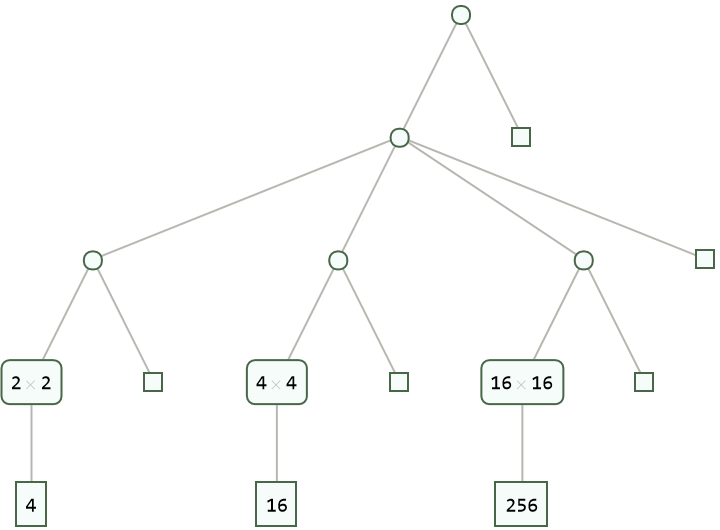### Scope (10)

Trace an empty evaluation chain:

 In:=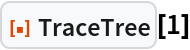Out=Trace a single step evaluation:

 In:=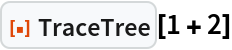Out=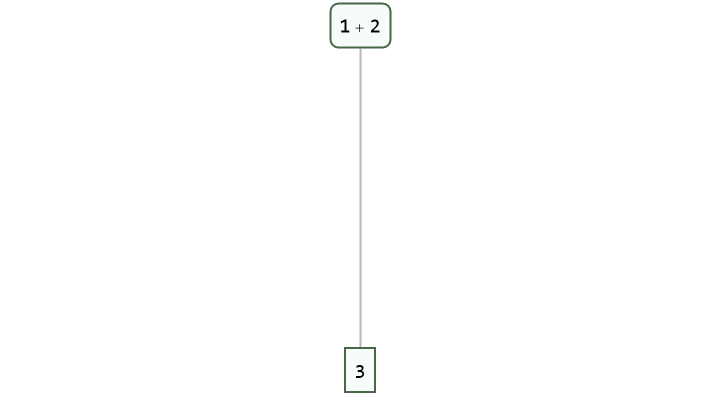Trace each branch in an evaluation:

 In:=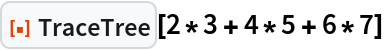Out=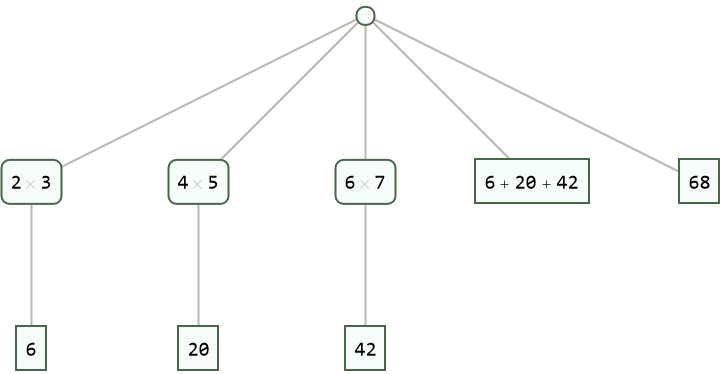Trace evaluations given by definitions:

 In:=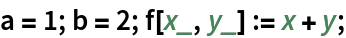In:=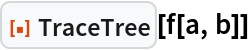Out=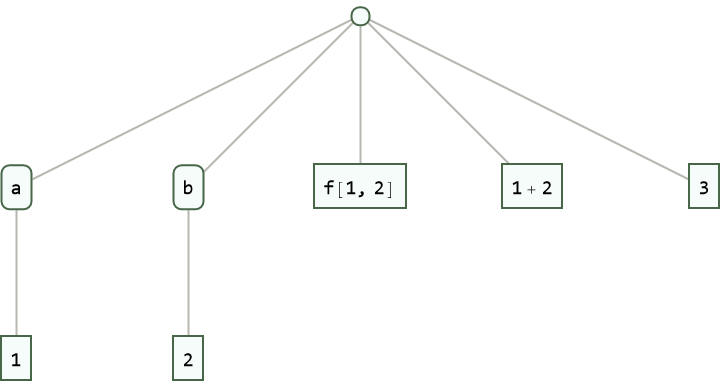Trace each step in an evaluation:

 In:=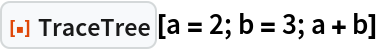Out=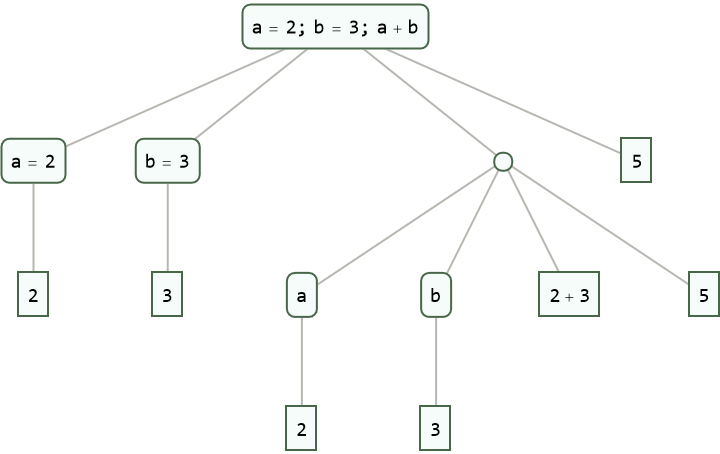Trace the operation of FoldList:

 In:=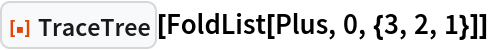Out=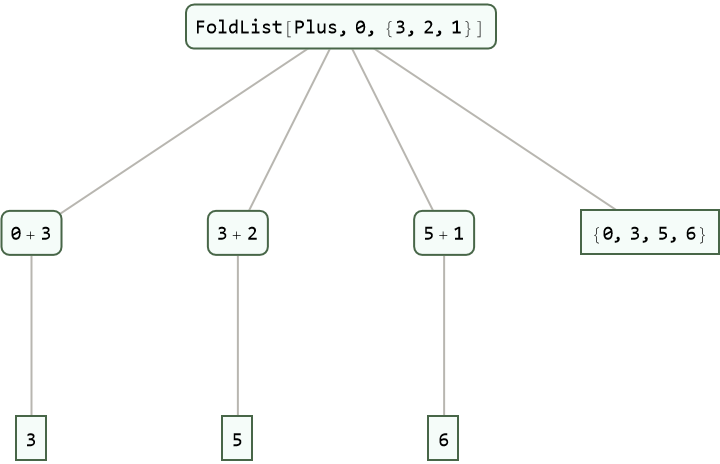Trace the steps in a non-standard evaluation:

 In:=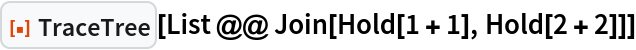Out=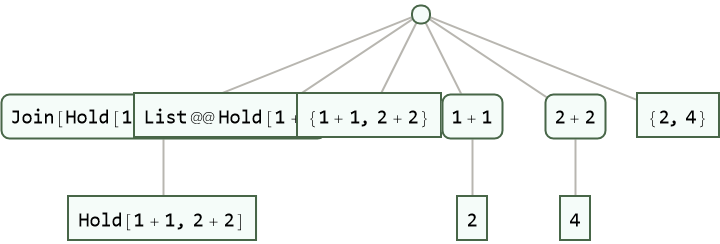Trace each step in an evaluation:

 In:=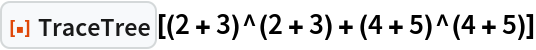Out=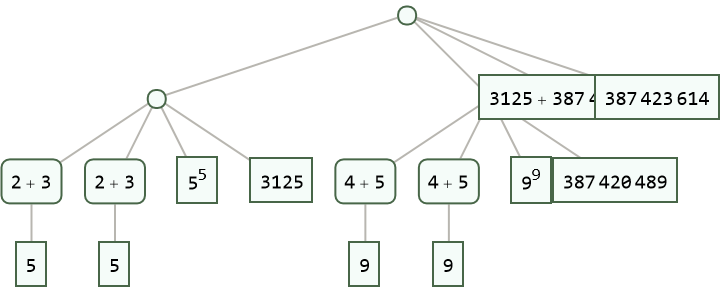Include only those expressions that match _Plus:

 In:=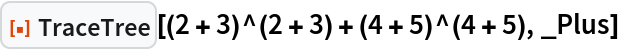Out=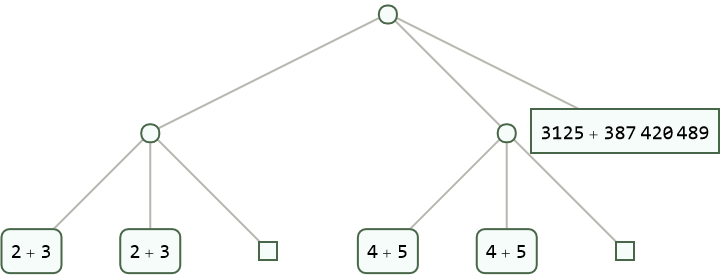Trace the computations with head Plus:

 In:=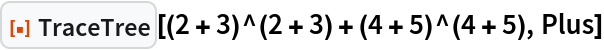Out=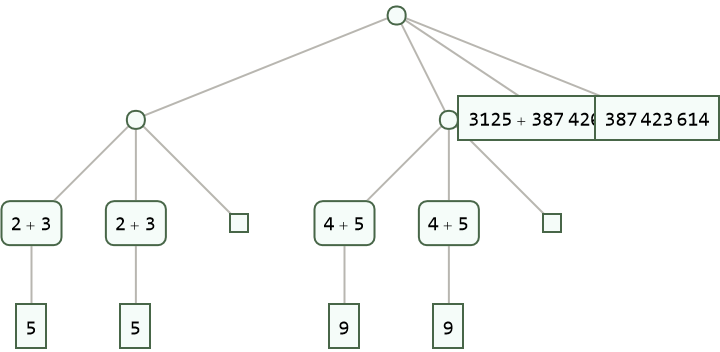Apply a transformation rule to expressions that match a pattern:

 In:=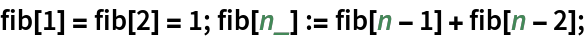In:=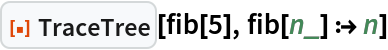Out=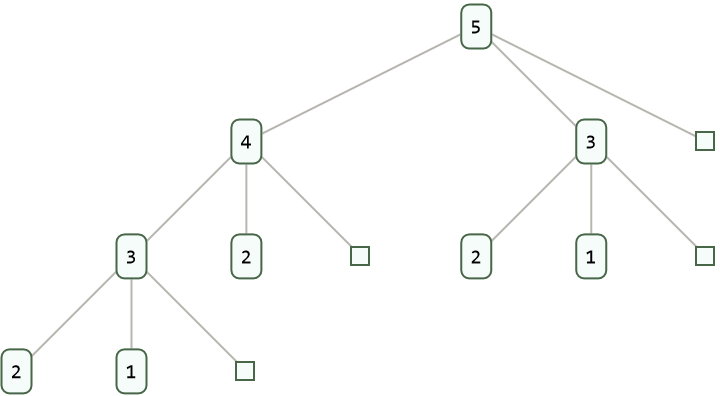Modify the setting for the form argument during the execution of TraceTree[expr,form] by resetting the value of the global variable \$TracePattern:

 In:=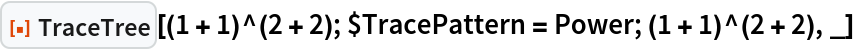Out=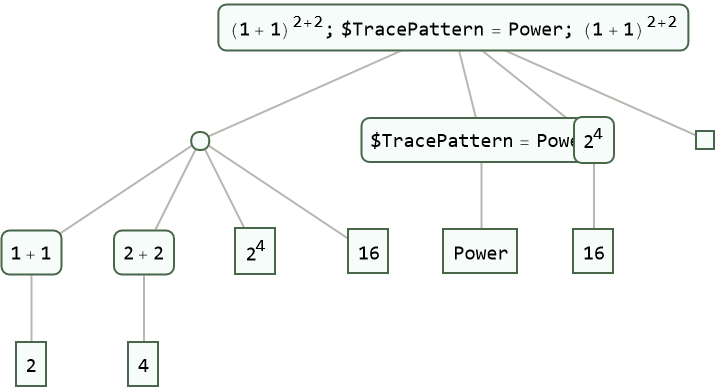### Options (23)

#### MatchLocalNames (2)

By default, symbols such as x match symbols with local names of the form x\$nnn:

 In:=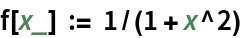In:=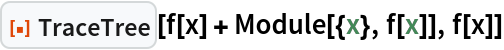Out=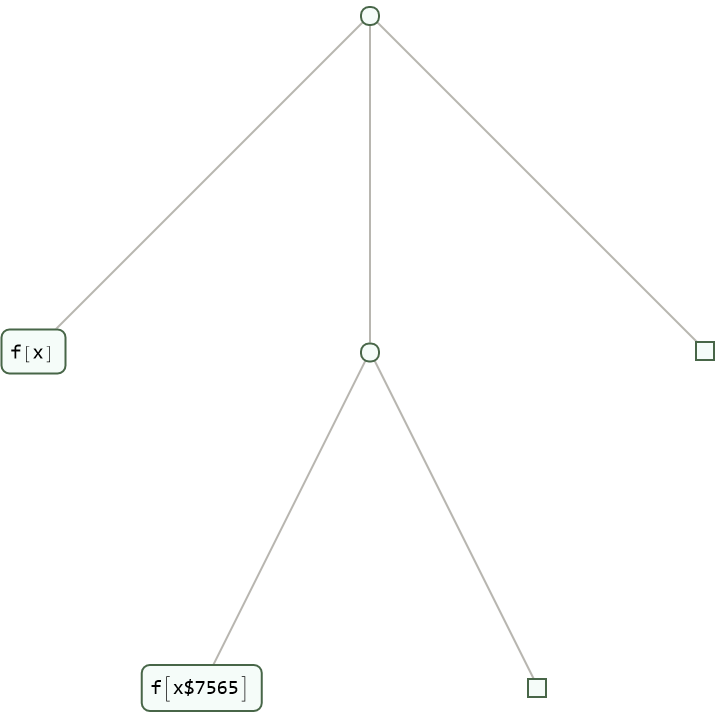With , only an explicit match of x will show up:

 In:=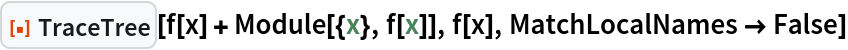Out=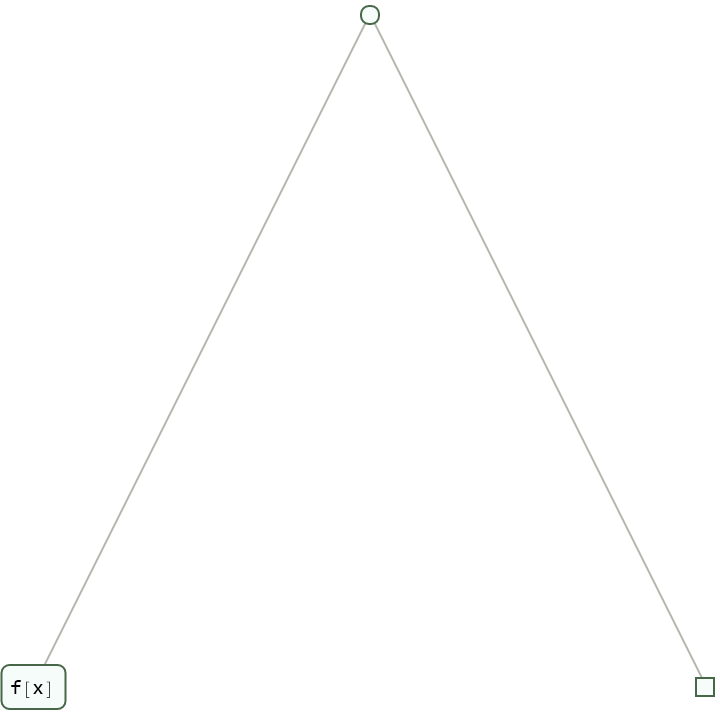#### TraceAbove (4)

A recursive definition for finding Fibonacci numbers:

 In:=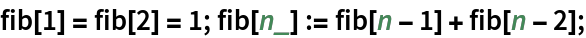Show only what sums of fib are encountered:

 In:=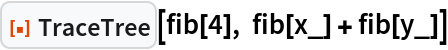Out=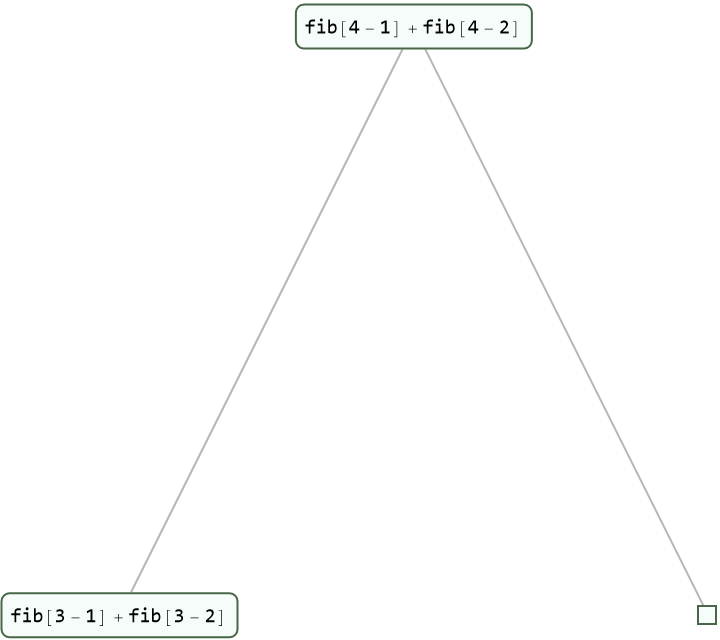Show the beginning of the evaluation chain that leads to each sum of fib:

 In:=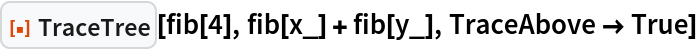Out=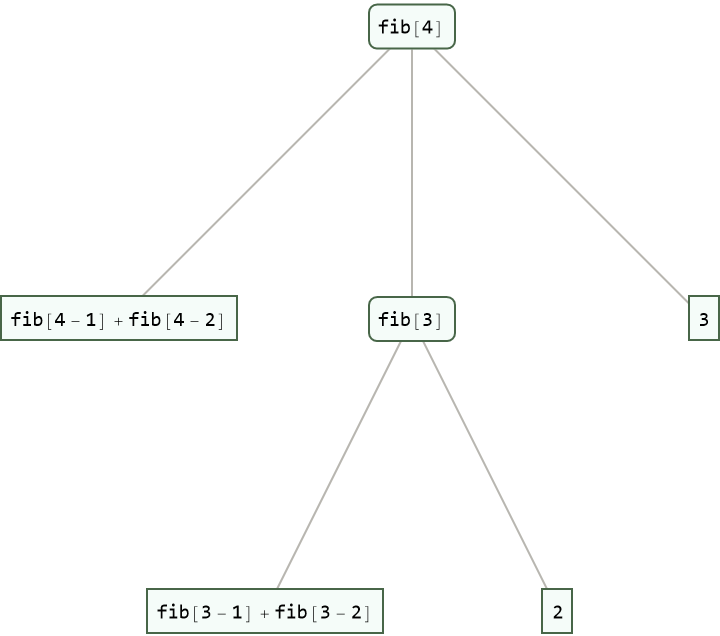Show the entire evaluation chain that leads to each sum of fib:

 In:=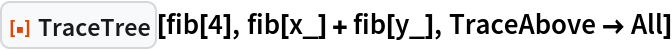Out=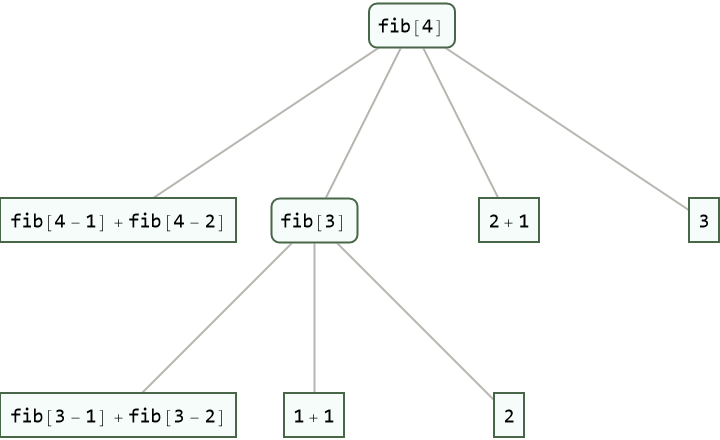#### TraceBackward (4)

A recursive definition for finding Fibonacci numbers:

 In:=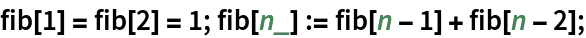Show only what additions of positive integers are required:

 In:=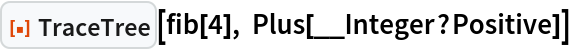Out=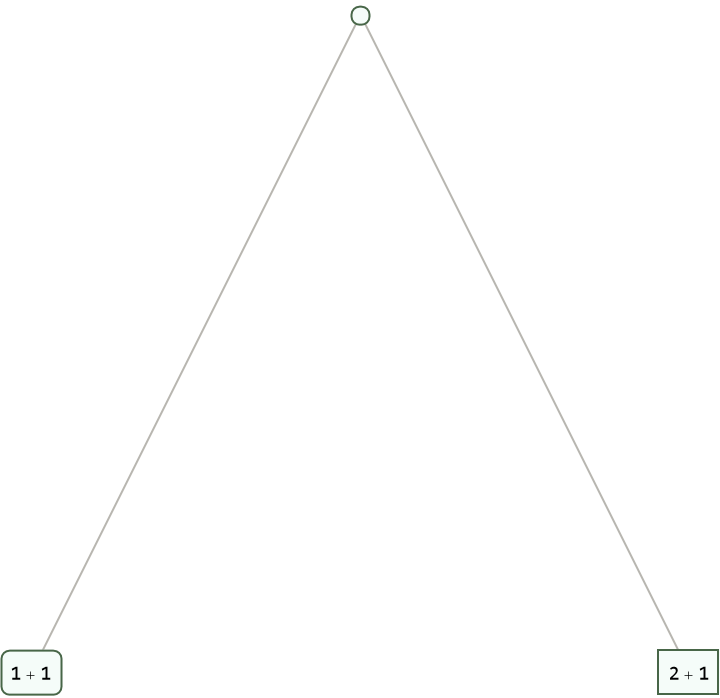Show the beginning of the evaluation chain that leads to each addition:

 In:=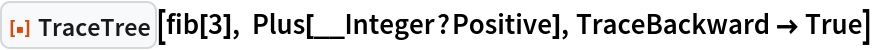Out=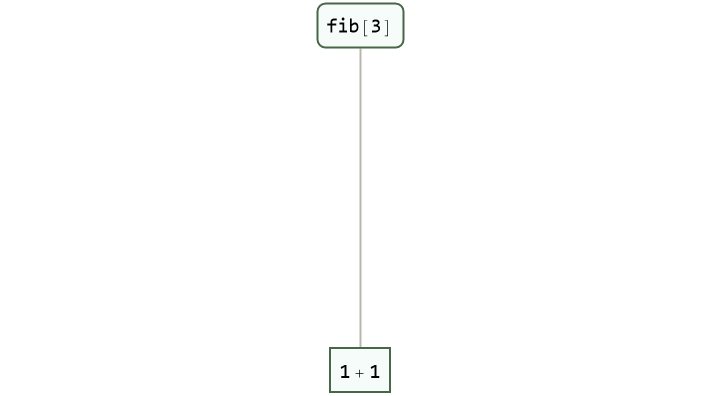Show all intermediate evaluations that led to each addition:

 In:=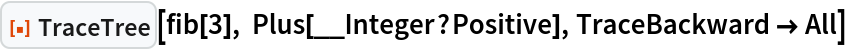Out=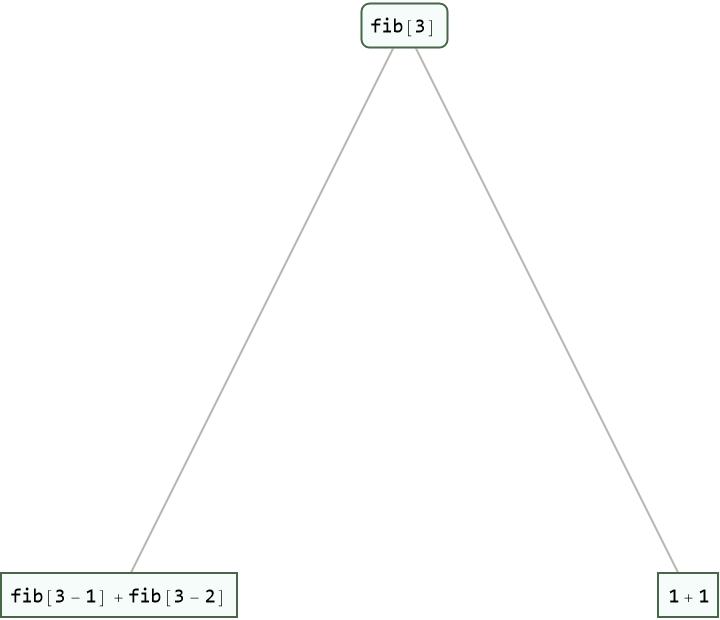#### TraceDepth (3)

A recursive definition for finding Fibonacci numbers:

 In:=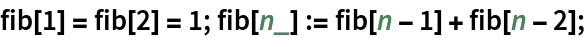Trace only evaluations through depth 3:

 In:=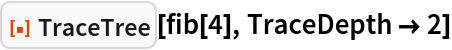Out=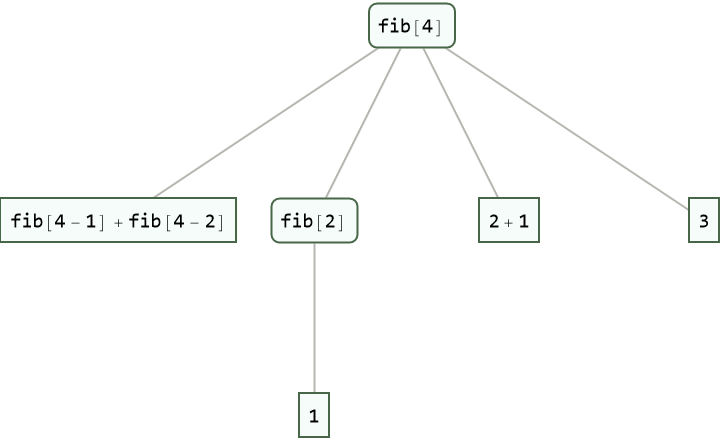Trace all evaluations:

 In:=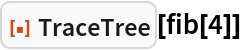Out=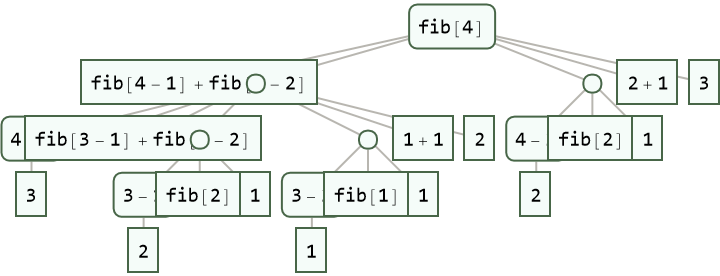#### TraceForward (4)

A recursive definition for finding Fibonacci numbers:

 In:=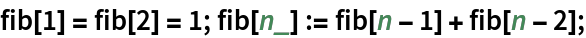Show only what evaluations of fib are encountered:

 In:=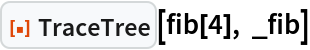Out=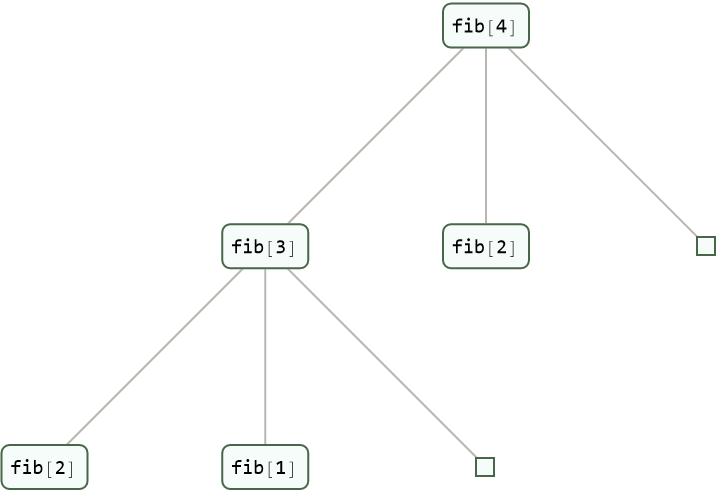Show only the evaluations of fib and the results:

 In:=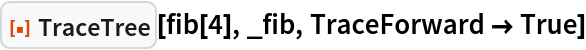Out=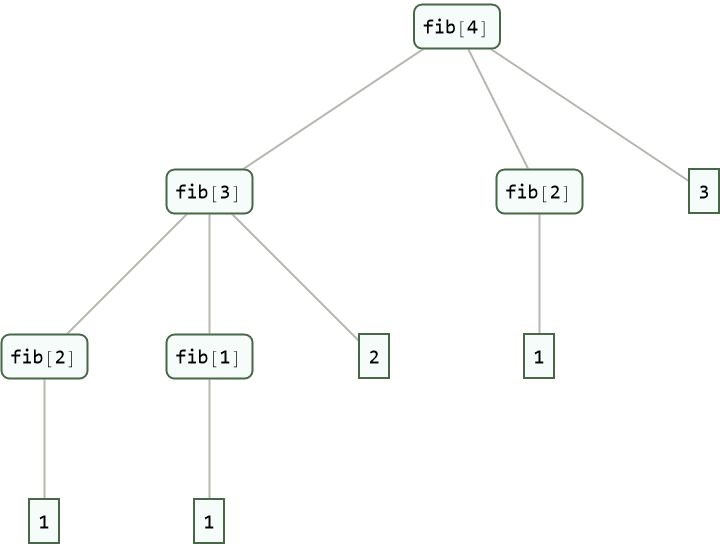Show all intermediate evaluations between calls of fib and the result:

 In:=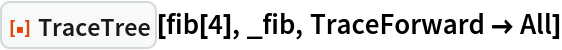Out=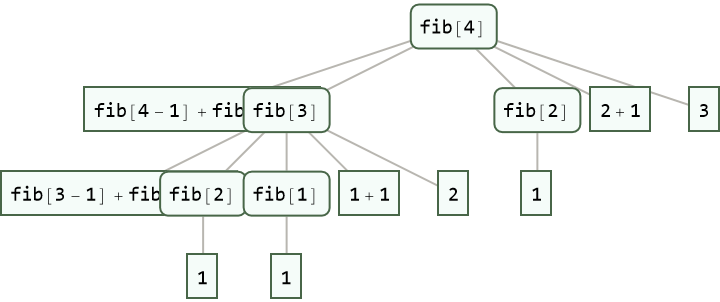#### TraceOff (2)

Trace evaluation of an expression that evaluates a function g:

 In:=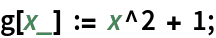In:=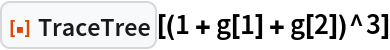Out=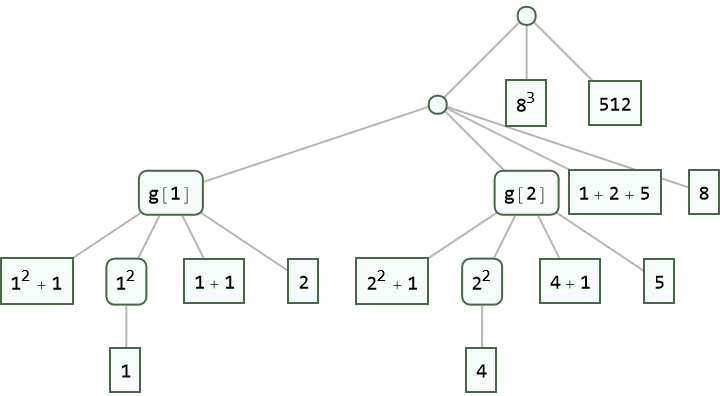Omit evaluations required to get the values of g:

 In:=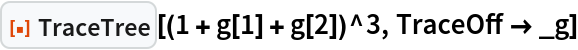Out=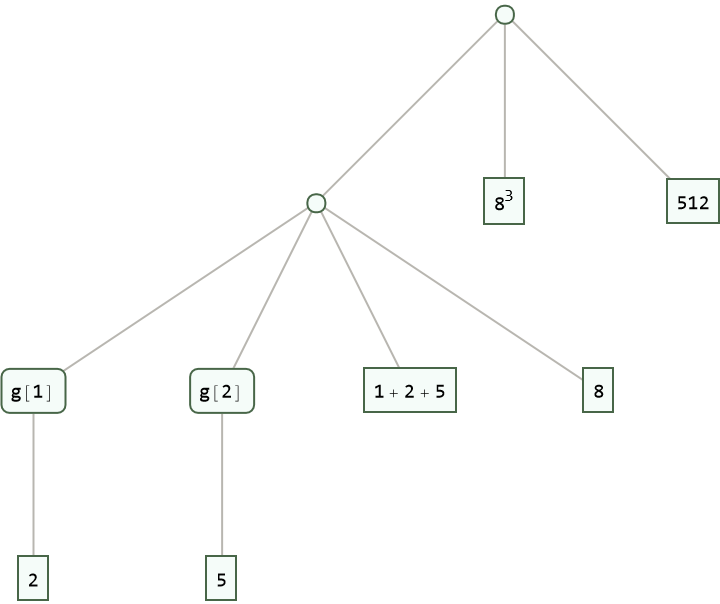#### TraceOn (2)

Trace evaluation of an expression that evaluates a function g:

 In:=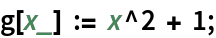In:=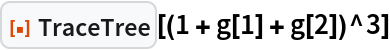Out=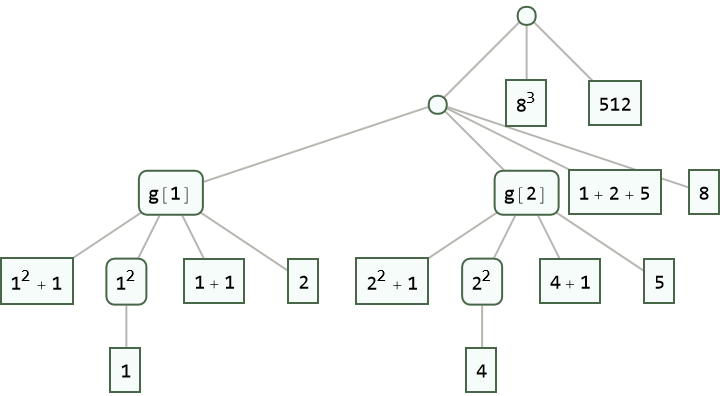Trace only evaluation inside of g:

 In:=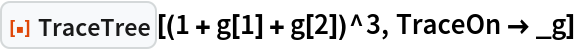Out=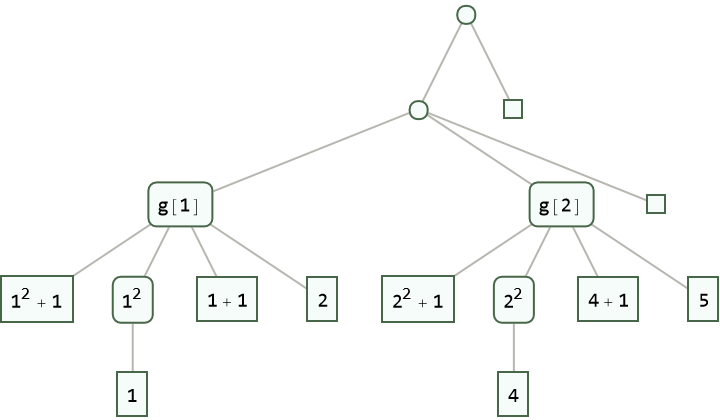#### TraceOriginal (2)

Trace evaluation of an expression showing evaluation chains for expressions that change:

 In:=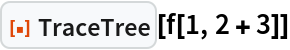Out=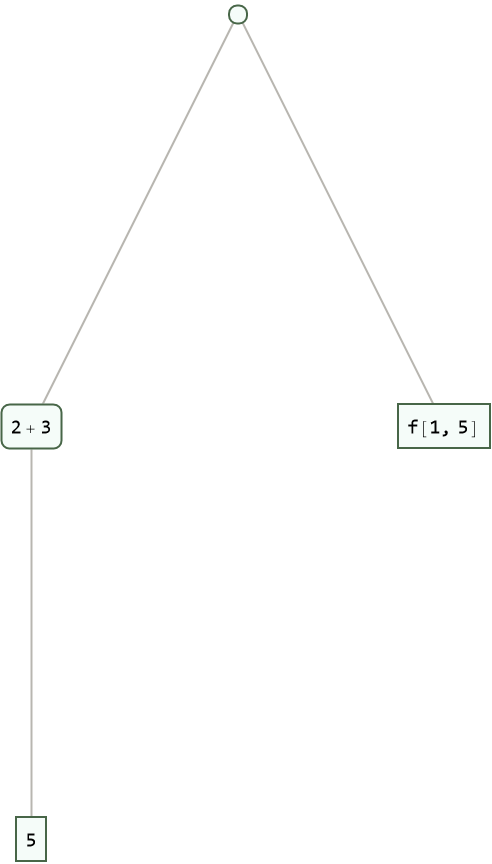Show evaluation chains even for expressions that do not change:

 In:=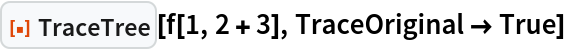Out=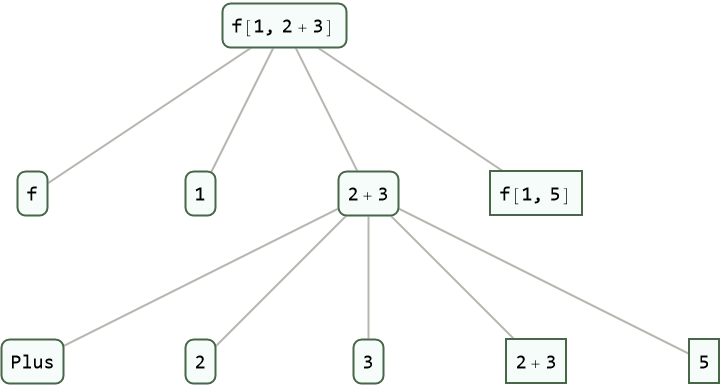### Properties and Relations (2)

TraceTree[expr] traces each step in the evaluation of expr:

 In:=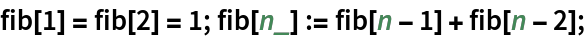In:=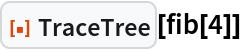Out=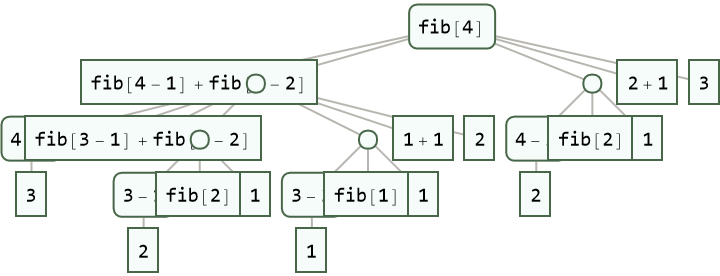TraceTree[expr,form] includes only those expressions that match form:

 In:=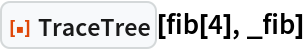Out=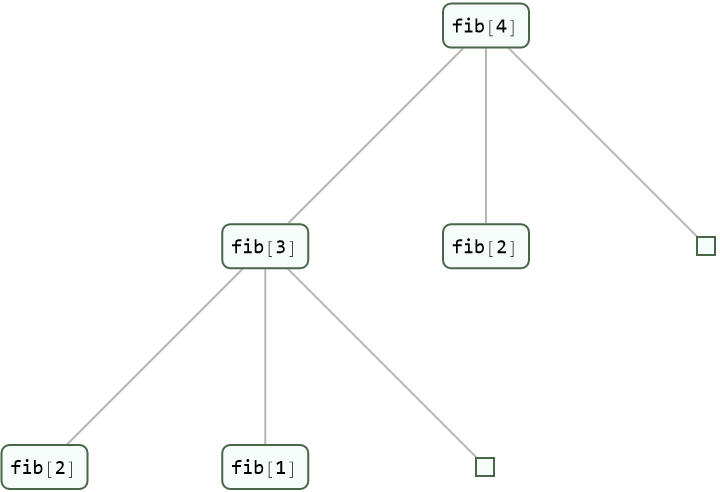This corresponds to deleting all expressions that do not match form, then deleting empty evaluation chains:

 In:=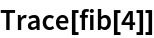Out=In:=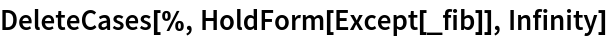Out=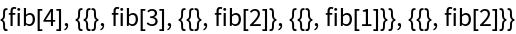In:=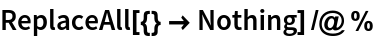Out=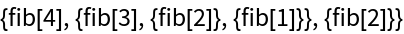In:=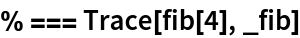Out=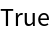All expressions in the tree returned by TraceTree are wrapped in HoldForm to prevent evaluation:

 In:=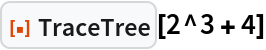Out=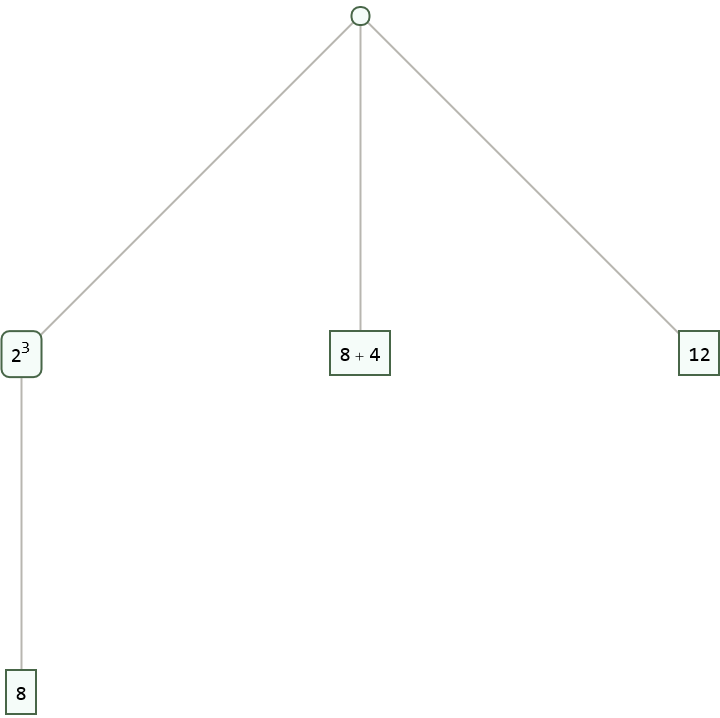## Version History

• 1.0.0 – 15 July 2022